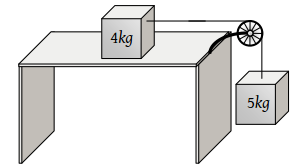Two masses of 4 kg and 5 kg are connected by a string passing through a frictionless pulley and are kept on a frictionless table as shown in the figure. The acceleration of 5 kg mass is(1) 49 m/s2

(2) 5.44 m/s2

(3) 19.5 m/s2

(4) 2.72 m/s2

Concept Videos :-

#7-Solved-Examples-1
#8-Solved-Examples-2
#9-Solved-Examples-3
#10-Solved-Examples-4
#11-Solved-Examples-5
#12-Solved-Examples-6

Concept Questions :-

Application of laws

(2) $a=\frac{{m}_{2}}{{m}_{1}+{m}_{2}}×g=\frac{5}{4+5}×9.8=\frac{49}{9}=5.44\text{\hspace{0.17em}}m/{s}^{2}$

Difficulty Level:

• 11%
• 70%
• 15%
• 6%